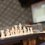# Nice geometry problem

Let $$ABC$$ be a triangle. $$D$$ and $$E$$ lie on $$AB$$ such that $$AD = AC, BE = BC$$ and the points $$D, A, B, E$$ are collinear in that order. The bisectors of angle $$A$$ and $$B$$ intersect $$BC, AC$$ at $$P$$ and $$Q$$ respectively, and the circumcircle of $$ABC$$ at $$M$$ and $$N$$ respectively. Let $$O_1$$ be the circumcenter of $$BME$$ and $$O_2$$ be the circumcenter of $$AND$$. $$AO_1$$ and $$BO_2$$ intersect at $$X$$. Prove that $$CX$$ is perpendicular to $$PQ$$.

Source : Serbia $2008$.Note by Zi Song Yeoh
6 years, 8 months ago

This discussion board is a place to discuss our Daily Challenges and the math and science related to those challenges. Explanations are more than just a solution — they should explain the steps and thinking strategies that you used to obtain the solution. Comments should further the discussion of math and science.

When posting on Brilliant:

• Use the emojis to react to an explanation, whether you're congratulating a job well done , or just really confused .
• Ask specific questions about the challenge or the steps in somebody's explanation. Well-posed questions can add a lot to the discussion, but posting "I don't understand!" doesn't help anyone.
• Try to contribute something new to the discussion, whether it is an extension, generalization or other idea related to the challenge.
• Stay on topic — we're all here to learn more about math and science, not to hear about your favorite get-rich-quick scheme or current world events.

MarkdownAppears as
*italics* or _italics_ italics
**bold** or __bold__ bold
- bulleted- list
• bulleted
• list
1. numbered2. list
1. numbered
2. list
Note: you must add a full line of space before and after lists for them to show up correctly
paragraph 1paragraph 2

paragraph 1

paragraph 2

[example link](https://brilliant.org)example link
> This is a quote
This is a quote
    # I indented these lines
# 4 spaces, and now they show
# up as a code block.

print "hello world"
# I indented these lines
# 4 spaces, and now they show
# up as a code block.

print "hello world"
MathAppears as
Remember to wrap math in $$ ... $$ or $ ... $ to ensure proper formatting.
2 \times 3 $2 \times 3$
2^{34} $2^{34}$
a_{i-1} $a_{i-1}$
\frac{2}{3} $\frac{2}{3}$
\sqrt{2} $\sqrt{2}$
\sum_{i=1}^3 $\sum_{i=1}^3$
\sin \theta $\sin \theta$
\boxed{123} $\boxed{123}$

## Comments

Sort by:

Top Newest

b=c square

- 6 years, 8 months ago

Log in to reply

Solution (SPOILER) :

We let $AB = c, BC = a, CA = b$ for simplicity.

Note that $AE \cdot AQ = (a + c) \cdot \frac{bc}{a + c} = bc$, by Angle Bisector Theorem. Also, $AP \cdot AM = AP \cdot (AP + PM) = AP^2 + AP \cdot PM = AP^2 + BP \cdot CP$, by Power of Point. Since by Stewart's Theorem, $AP^2 + BP \cdot CP = \frac{AB^2 \cdot CP + AC^2 \cdot BP}{BC} = \frac{c^2(\frac{ab}{b + c}) + b^2(\frac{ac}{b + c})}{a} = \frac{bc(b + c)}{b + c} = bc$, we have $AP \cdot AM = bc$.

Consider the following transformation (commonly known as $\sqrt{bc}$-inversion.) :

1) Reflect every point $X$ across the angle bisector of $\angle BAC$.

2) Invert about point $A$ with radius $\sqrt{bc}$.

So, it follows immediately that $E$ and $Q$ map to each other. Similarly, $P$ and $M$ map to each other. Thus, the circumcircle of $BME$ is mapped to the circumcircle of $CPQ$, with center $O$. Therefore, $AO$ is isogonal to $AO_1$ in $\angle BAC$, since the reflection of $O_1$ across the angle bisector of $\angle BAC$ is collinear with $A$ and $O$. Similarly, $BO$ is isogonal to $BO_2$ in $\angle ABC$. Thus, $O$ and $X$ are isogonal conjugates with respect to $\triangle ABC$. Finally, since in triangle $CQP$, $CX$ is isogonal with $CO$, $C, H, X$ are collinear and thus $CX \perp PQ$. ($H$ is the orthocenter of $CPQ$)

The last line follows from the fact that $O$ and $H$ are isogonal conjugates.

- 6 years, 8 months ago

Log in to reply

An easy way to establish $AP\cdot AM=bc$: we have $\angle BAM=\angle CAP$ (since $AP$ angle bisector) and $\angle ACB=\angle AMB$ since $ABMC$ cyclic. It follows that $\triangle ABM\sim \triangle APC$ so side ratios are equal.

- 6 years, 8 months ago

Log in to reply

Can you provide a diagramatical representation . I coudnt.draw a figure for the given information

- 6 years, 8 months ago

Log in to reply

(a)=ad bxqpxmn-1-1X1x(a)(B)2x

- 6 years, 8 months ago

Log in to reply

I m not able to figure out the diagram. Can someone help please?

- 6 years, 8 months ago

Log in to reply

Who can prove 4=5

- 6 years, 8 months ago

Log in to reply

×

Problem Loading...

Note Loading...

Set Loading...